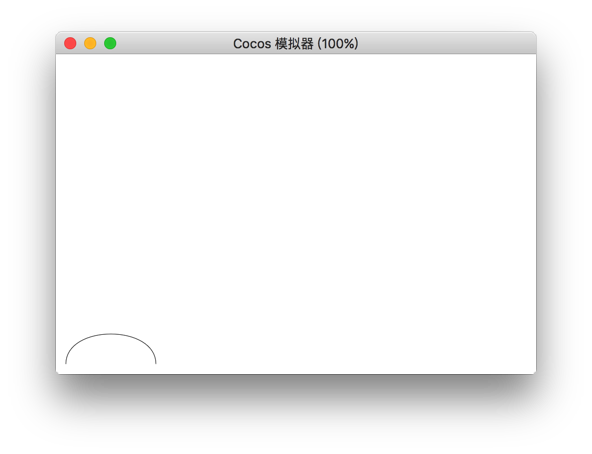# Bezier Curves

The `bezierCurveTo()` method adds a bezier curve to the current path by using the specified control point that can generate the cubic bezier curve.

Note: Cubic Bezier curves require three control points. The first two points are for the control points in the cubic Bezier calculation, and the third point is the end point of the curve. The starting point of the curve is the last point in the current path.

Parameter Description
cp1x The x coordinate of the first bezier control point.
cp1y The y coordinate of the first bezier control point.
cp2x The x coordinate of the second Bezier control point.
cp2y The x coordinate of the second Bezier control point.
x The x coordinate of the end point.
y The y coordinate of the end point.

## Example

``````const ctx = node.getComponent(Graphics);
ctx.moveTo(20,20);
ctx.bezierCurveTo(20,100,200,100,200,20);
ctx.stroke();
``````Return to the Graphics Component Reference documentation.